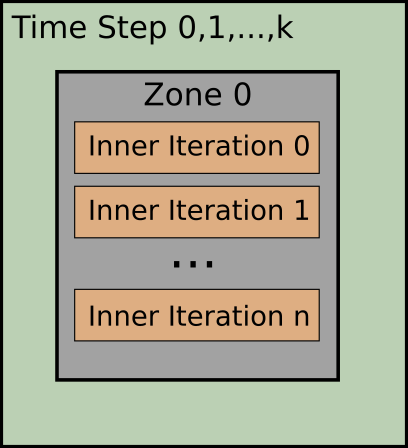# Solver Setup

This guide is for version 7 only

This is a basic introduction on how to set up a simulation using SU2. We distinguish between single-zone computations and multi-zone computations. The following considers a single zone only. For an explanation on multi-zone problems, continue with Basics of Multi-Zone Computations.

# Defining the Problem

SU2 is capable of dealing with different kinds of physical problems. The kind of problem is defined by choosing a solver using the `SOLVER` option. A list of possible values and a description can be found in the following table:

Option Value Problem Type
`EULER` Euler’s equation Finite-Volume method
`NAVIER_STOKES` Navier-Stokes’ equation Finite-Volume method
`RANS` Reynolds-averaged Navier-Stokes’ Finite-Volume method
`INC_EULER` Incompressible Euler’s equation Finite-Volume method
`INC_NAVIER_STOKES` Incompressible Navier-Stokes’ Finite-Volume method
`INC_RANS` Incompressible Reynolds-averaged Navier-Stokes’ Finite-Volume method
`HEAT_EQUATION_FVM` Heat equation Finite-Volume method
`ELASTICITY` Equations of elasticity Finite-Element method
`FEM_EULER` Euler’s equation Discontinuous Galerkin FEM
`FEM_NAVIER_STOKES` Navier-Stokes’ equation Discontinuous Galerkin FEM
`MULTIPHYSICS` Multi-zone problem with different solvers in each zone -

Every solver has its specific options and we refer to the tutorial cases for more information. However, the basic controls detailed in the remainder of this page are the same for all problems.

## Restarting the simulation

A simulation can be restarted from a previous computation by setting `RESTART_SOL=YES`. If it is a time-dependent problem, additionally `RESTART_ITER` must be set to the time iteration index you want to restart from:

``````% ------------------------- Solver definition -------------------------------%
%
% Type of solver
SOLVER= EULER
%
% Restart solution (NO, YES)
RESTART_SOL= NO
%
% Iteration number to begin unsteady restarts (used if RESTART_SOL= YES)
RESTART_ITER= 0
%
``````

# Controlling the simulation

A simulation is controlled by setting the number of iterations the solver should run (or by setting a convergence critera). The picture below depicts the two types of iterations we consider.SU2 makes use of an outer time loop to march through the physical time, and of an inner loop which is usually a pseudo-time iteration or a (quasi-)Newton scheme. The actual method used depends again on the specific type of solver.

## Time-dependent Simulation

To enable a time-dependent simulation set the option `TIME_DOMAIN` to `YES` (default is `NO`). There are different methods available for certain solvers which can be set using the `TIME_MARCHING` option. For example for any of the FVM-type solvers a first or second-order dual-time stepping (`DUAL_TIME_STEPPING-1ST_ORDER`/`DUAL_TIME_STEPPING-2ND_ORDER`) method or a conventional time-stepping method (`TIME_STEPPING`) can be used.

``````% ------------------------- Time-dependent Simulation -------------------------------%
%
TIME_DOMAIN= YES
%
% Time Step for dual time stepping simulations (s)
TIME_STEP= 1.0
%
% Total Physical Time for dual time stepping simulations (s)
MAX_TIME= 50.0
%
% Number of internal iterations
INNER_ITER= 200
%
% Number of time steps
TIME_ITER= 200
%
``````

The solver will stop either when it reaches the maximum time (`MAX_TIME`) or the maximum number of time steps (`TIME_ITER`), whichever event occurs first. Depending on the `TIME_MARCHING` option, the solver might use an inner iteration loop to converge each physical time step. The number of iterations within each time step is controlled using the `INNER_ITER` option.

## Steady-state Simulation

A steady-state simulation is defined by using `TIME_DOMAIN=NO`, which is the default value if the option is not present. In this case the number of iterations is controlled by the option `ITER`.

Note: To make it easier to switch between steady-state, time-dependent and multizone simulations, the option `INNER_ITER` can also be used to specify the number of iterations. If both options are present, `INNER_ITER` has precedence.

## Setting convergence criteria

Despite setting the maximum number of iterations, it is possible to use a convergence criterion so that the solver will stop when it reaches a certain value of a residual or if variations of a coefficient are below a certain threshold. To enable a convergence criterion use the option `CONV_FIELD` to set an output field that should be monitored. The list of possible fields depends on the solver. Take a look at Custom Output to learn more about output fields. Depending on the type of field (residual or coefficient) there are two types of methods:

### Residual

If the field set with `CONV_FIELD` is a residual, the solver will stop if it is smaller than the value set with `CONV_RESIDUAL_MINVAL` option. Example:

``````% ------------------- Residual-based Convergence Criteria -------------------------%
%
CONV_FIELD= RMS_DENSITY
%
%
% Min value of the residual (log10 of the residual)
CONV_RESIDUAL_MINVAL= -8
%
``````

### Coefficient

If the field set with `CONV_FIELD` is a coefficient, a Cauchy series approach is applied. A Cauchy element is defined as the difference of the coefficient between two consecutive iterations. The solver will stop if the sum over a certain number of elements (set with `CONV_CAUCHY_ELEMS`) is smaller than the value set with `CONV_CAUCHY_EPS`. The current value of the Cauchy coefficient can be written to screen or history by adding the `CAUCHY` field to the `SCREEN_OUTPUT` or `HISTORY_OUTPUT` option (see Custom Output). Example:

``````% ------------------ Coefficient-based Convergence Criteria -----------------------%
%
CONV_FIELD= DRAG
%
%
% Number of elements to apply the criteria
CONV_CAUCHY_ELEMS= 100
%
% Epsilon to control the series convergence
CONV_CAUCHY_EPS= 1E-10
%
``````

For both methods the option `CONV_STARTITER` defines when the solver should start monitoring the criterion.